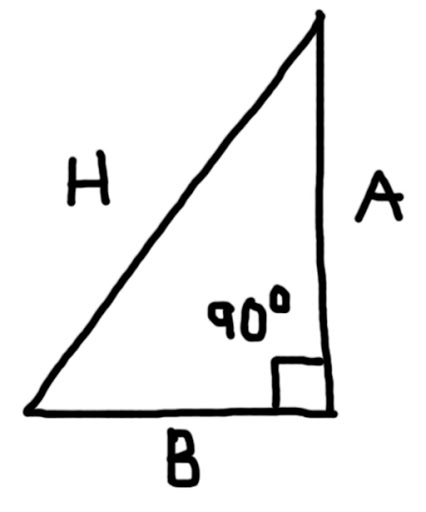## Thursday, October 11, 2012

### Calculator Tricks - Part 3

Welcome to Part 3 of the Calculator Trick series using the Simple Calculator. This part covers:

* Squaring Numbers
* Reciprocals
* Geometric Applications

Just a reminder, a simple calculator has the following functions: arithmetic (+, -, ×, ÷), square root (√), percent (%), and memory (M+, M-, MR, MC). If your calculator has a MRC clear, this means press MRC once to recall memory, twice to clear it.

Squaring Numbers

---------------
To square a number, use the following key sequence:

× =
--------------
Example 1: 7^2

Keystrokes:
7 × =
---------------
Example 2: √(3^2 + 4^2) = 5

Use the memory keys to solve this problem. Squaring a number is multiplying a number by itself. Apply the square root last.

Keystrokes: MC (Again, always a good idea to clear memory to start)
3 × = M+ (3^2 = 9)
4 × = M+ (4^2 = 16)
MR (3^2 + 4^2 = 25)
(√25 = 5)
----------------

Reciprocals

---------------
To find the reciprocal of a number, use the following key sequence:

÷ ÷ =
(Yes, press the division key twice)
---------------
Example 1: 1/7 ≈ 0.142857

Keystrokes: 7 ÷ ÷ =
---------------
Example 2: 1/(1/5 + 1/3.2) ≈ 1.9512195

Strategy: Tackle the denominator first. Of course, anytime you have mixed operations, chances are you will be using the memory register.

Keystrokes:
MC
5 ÷ ÷ = M+ (1/5 = 0.2)
3.2 ÷ ÷ = M+ (adding 1/3.2 = 0.3125)
MR (1/5 + 1/3.2 = 0.5125 )
÷ ÷ = ( 1/(1.5 + 1/3.2) ≈ 1.9512195)
---------------

Geometric Applications

Area of the a Circle:
A = π r^2

where r is the radius and π is the constant pi. In this series, I am working with an 8 digit calculator, I will use the approximation π ≈ 3.1415927. I can use less digits, but I want as much accuracy as possible.

---------------
Area of a Circle: A = π r^2

Keystrokes: radius × = × 3.1415927 =
---------------
Example: Find an area of a circle with a radius of 14.5 inches.

Keystrokes: 14.5 × = × 3.1415927 =

The area is approximately 660.51986 square inches.
---------------

Distance between two Cartesian points (x1, y1) and (x2, y2):
√((x2 - x1)^2 + (y2 - y1)^2)

---------------
Distance between (x1, y1) and (x2, y2):

Keystrokes: MC
x2 - x1 = × = M+
y2 - y1 = × = M+
MR √

---------------
Example: Find the distance between the points (5,6) and (1,9).

Note: (x1, y1) = (5, 6) and (x2, y2) = (1, 9)

Keystrokes: MC
1 - 5 = × = M+
(Display: 16 M)
9 - 6 = × = M+ (Display: 9 M)
MR √ (Display: 5 M)

The distance between the points (5, 6) and (1, 9) is 5 units.
---------------

Right TrianglesArea = 1/2 × A × B

Pythagorean Theorem: H^2 = A^2 + B^2

---------------
With A = 3.9 inches, B = 2.4 inches. Find the area and the hypotenuse (H).

Area:
Keystrokes: 3.9 × 2.4 ÷ 2 =

The area is 4.68 square inches.

Finding the Hypotenuse:
Keystrokes: MC 3.9 × = M+
2.4 × = M+
MR √

The hypotenuse is approximately 4.5793012 inches.
---------------

Next time in Part 4, we will cover shopping and short-term loans with simple interest.

Eddie

This blog is property of Edward Shore, 2012.